JEE  >  BITSAT Logical Reasoning Test - 7

# BITSAT Logical Reasoning Test - 7

Test Description

## 10 Questions MCQ Test BITSAT Mock Tests Series & Past Year Papers | BITSAT Logical Reasoning Test - 7

BITSAT Logical Reasoning Test - 7 for JEE 2022 is part of BITSAT Mock Tests Series & Past Year Papers preparation. The BITSAT Logical Reasoning Test - 7 questions and answers have been prepared according to the JEE exam syllabus.The BITSAT Logical Reasoning Test - 7 MCQs are made for JEE 2022 Exam. Find important definitions, questions, notes, meanings, examples, exercises, MCQs and online tests for BITSAT Logical Reasoning Test - 7 below.
Solutions of BITSAT Logical Reasoning Test - 7 questions in English are available as part of our BITSAT Mock Tests Series & Past Year Papers for JEE & BITSAT Logical Reasoning Test - 7 solutions in Hindi for BITSAT Mock Tests Series & Past Year Papers course. Download more important topics, notes, lectures and mock test series for JEE Exam by signing up for free. Attempt BITSAT Logical Reasoning Test - 7 | 10 questions in 10 minutes | Mock test for JEE preparation | Free important questions MCQ to study BITSAT Mock Tests Series & Past Year Papers for JEE Exam | Download free PDF with solutions
 1 Crore+ students have signed up on EduRev. Have you?
BITSAT Logical Reasoning Test - 7 - Question 1

### Choose the word which is least like the other words in the group .

BITSAT Logical Reasoning Test - 7 - Question 2

### Complete the analogous pair. Brick : Building :: ? : Letter

BITSAT Logical Reasoning Test - 7 - Question 3

### Group the following figures into three classes on the basis of identical properties.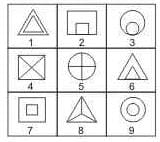Detailed Solution for BITSAT Logical Reasoning Test - 7 - Question 3

1,7,9 contain two similar elements one inside the other but not touching each other.
2,3,6 contain two similar elements one inside the other and both touching each ohter.
4,5,8 are divided into equal parts by straight lines emerging from the centre.

BITSAT Logical Reasoning Test - 7 - Question 4

Find the missing character.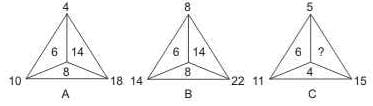Detailed Solution for BITSAT Logical Reasoning Test - 7 - Question 4

In fig. (A), 10 - 4 = 6, 18 - 10 = 8, 18 - 4 = 14.
In fig. (B), 14 - 8 = 6, 22 - 14 = 8, 22 - 8 = 14.
In fig. (C), 11 - 5 = 6, 15 - 11 = 4.
So, missing number = 15 - 5 = 10.

BITSAT Logical Reasoning Test - 7 - Question 5

Find out the term in the number series which is wrong.
0, 3, 8, 15, 24, 33

Detailed Solution for BITSAT Logical Reasoning Test - 7 - Question 5

The correct sequence is + 3, + 5, + 7, + 9, + 11.
So, 33 is wrong and must be replaced by (24 + 11)  i.e . 35.

BITSAT Logical Reasoning Test - 7 - Question 6

In each question, three sequences of letters/numerals are given which correspond to each other. Find out the letters/numerals that come in vacant places.
_ A D A C B _ _ B D C C
1 3 _ _ 1 2 4 2 _ _ _ _
a _ _ b _ _ c d ? ? ? ?

BITSAT Logical Reasoning Test - 7 - Question 7

Select the correct mirror image of the figure (X) from amongst the given alternatives.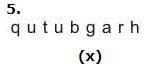BITSAT Logical Reasoning Test - 7 - Question 8

Select the correct mirror image of the figure (X) from amongst the given alternatives.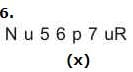BITSAT Logical Reasoning Test - 7 - Question 9

Each of the following questions consists of five Problem figures marked 1,2,3,4 and 5 followed by five Answer figures marked A,B,C,D and E . Select a figure from the Answer figures which will continue the same series as given in the Problem figures.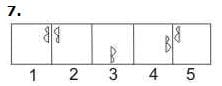BITSAT Logical Reasoning Test - 7 - Question 10

Each of the following questions consists of five Problem figures marked 1,2,3,4 and 5 followed by five Answer figures marked A,B,C,D and E . Select a figure from the Answer figures which will continue the same series as given in the Problem figures.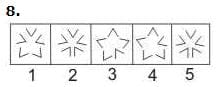## BITSAT Mock Tests Series & Past Year Papers

3 videos|15 docs|70 tests
 Use Code STAYHOME200 and get INR 200 additional OFF Use Coupon Code
Information about BITSAT Logical Reasoning Test - 7 Page
In this test you can find the Exam questions for BITSAT Logical Reasoning Test - 7 solved & explained in the simplest way possible. Besides giving Questions and answers for BITSAT Logical Reasoning Test - 7, EduRev gives you an ample number of Online tests for practice

## BITSAT Mock Tests Series & Past Year Papers

3 videos|15 docs|70 tests